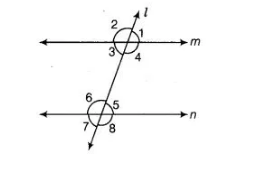# In the figure, ∠1 = 60° and ∠6 = 120°.`
Question:

In the figure, ∠1 = 60° and ∠6 = 120°. Show that the lines m and n are parallel.Solution:

Given In the figure ∠1 = 60° and ∠6 = 120°

To show m||n

Proof Since, ∠1 = 60° and ∠6 = 120°

Here, ∠1 = ∠3 [vertically opposite angles]

∠3 = ∠1 = 60°

Now, ∠3 + ∠6 = 60° + 120°

=> ∠3 + ∠6 = 180°

We know that, if the sum of two interior angles on same side of l is 180°, then lines are parallel.

Hence, m || n